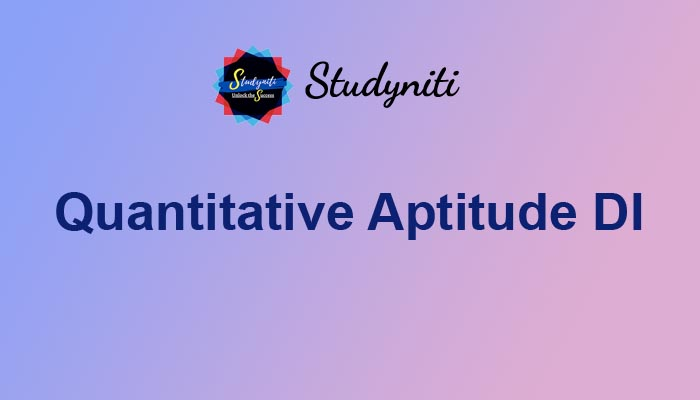## Payment Processing...# Quantitative Aptitude DI - Oct 23 2020

Here we are providing new series of Quantitative Aptitude Questions for upcoming exams, so the aspirants can practice it on a daily basis.

Study the following information carefully and answer the given questions?

Person A, B and C started a business by investing Rs. 20000, Rs. 30000 and Rs. __(a)__ respectively. After 5 months, A invested Rs. 10000 more but C withdraw Rs. 5000. And after another 4 months, B left the business. The share of A, B and C, at the end of the year is in the ratio of 62: 54: 113. Person M invested the amount, which is equal to the initial investment of C, in a certain scheme, which offers a simple interest at the rate of __(b)___ % per annum for 4 years. He earned a total amount of Rs. 74000 at the end of 4 years. There are 7 consecutive even and 5 consecutive odd numbers. The average of 7 consecutive even numbers is equal to 3b. The smallest number of 5 odd numbers is 3 less than the (8/7)th of ___(c)___. The product of second largest even number and the average of 5 consecutive odd number is 1960. The present age of father is five times the present age of his son. The average present age of father, son and daughter is 20 years. The age of father after 2 years is equal to “c”. Multiples of present age of mother and daughter is 432 years. The age of mother, after 8 years will be ___(d)____ years. Person P can do a piece of work in “d” days while P and Q together can complete the same piece of work in 14 2/3 days. Person Q can do the (7/11)th of the work in ___(e)___ days.

1) What value should come in place of “(a)”?

a) 40000

b) 60000

c) 35000

d) 50000

e) None of these

2) What value should come in place of “(b)”?

a) 12

b) 10

c) 8

d) 15

e) None of these

3) What value should come in place of “(c)”?

a) 44

b) 46

c) 42

d) 48

e) None of these

4) What value should come in place of “(d)”?

a) 50

b) 42

c) 46

d) 44

e) None of these

5) What value should come in place of “(e)”?

a) 12

b) 14

c) 18

d) 16

e) None of these

Directions (Q. 1 - 5):

The share of A, B and C,

= > [20000 * 5 + 30000 * 7] : [30000 * 9] : [a * 5 + (a - 5000) * 7]

= > [100000 + 210000] :  : [5a + 7a - 35000]

= >  :  : [12a - 35000]

According to the question,

(270000) / (12a - 35000) = 54 / 113

12a - 35000 = 565000

12a = 600000

a = 50000

The value of “a” be Rs. 50000

The initial investment of C = Rs. 50000

The principal amount invested by person M = Rs. 50000

SI = PNR / 100

Let the rate of interest be “b”,

According to the question,

50000 + [(50000 * b * 4) / 100] = 74000

[(50000 * b * 4) / 100] = 74000 - 50000

b = (24000 * 100) / (50000 * 4) = 12

The value of “b” be 12

The average of 7 consecutive even numbers = 3b = 36

The average of consecutive number/odd/even number is always the middle number. So, the numbers are,

= > 30, 32, 34, 36, 38, 40, 42

The second largest even number = 40

Let the average of 5 odd number be xm

The product of second largest even number and the average of 5 consecutive odd number = 1960

40 * x = 1960

x = 1960 / 40 = 49

The five consecutive odd numbers are,

45, 47, 49, 51, 53

The smallest odd number = (8/7)* c - 3

45 + 3 = (8/7) * c

c = 48 * (7/8) = 42

The value of “c” be 42

The present age of father and son is in the ratio = 5 : 1

The age of father after 2 years = “c” = 42

The present age of father = 40 years

The present age of son = (40 / 5) * 1 = 8 years

The average present age of father, son and daughter = 20 years

40 + 8 + Daughter’s present age = 60

Daughter’s present age = 60 - 48 = 12 years

Multiples of present age of mother and daughter = 432 years

Mother * 12 = 432

Mother’s present age = (432 / 12) = 36 years

The age of mother, after 8 years = 36 + 8 = 44 years

The value of “d” be 44 years

Person P can do a piece of work = 44 days

(P + Q) can complete the work in, = 44/3 days

Q’s 1 day work = (3/44) - (1/44) = 2/44 = 1/22

Q can complete the whole work in, 22 days.

Person Q can complete (7/11)th of the work in, = 22 * (7/11) = 14 days

The value of “e” be 14

1) Answer: D

The value of “a” be Rs. 50000

2) Answer: A

The value of “b” be 12

3) Answer: C

The value of “c” be 42

4) Answer: D

The value of “d” be 44

5) Answer: B

The value of “e” be 14